# 1741 What Do You Notice About the Remainders?

### Today’s Puzzle:

The table below is a list of the first 280 prime numbers. Below each prime number is its remainder when the prime number is divided by 6. What do you notice about the remainders? What do you wonder?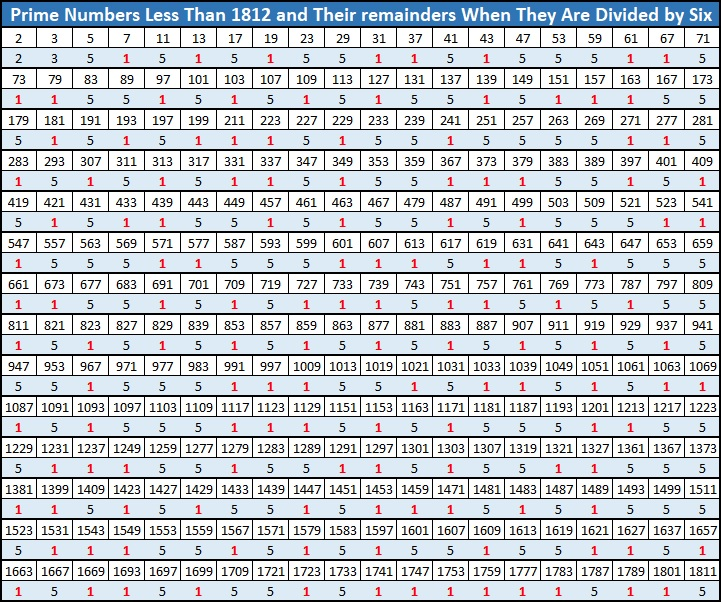Did you notice a fun fact starting with the number 1741? I would like to thank OEIS.org for making me aware of it.

### Factors of 1741:

• 1741 is a prime number.
• Prime factorization: 1741 is prime.
• 1741 has no exponents greater than 1 in its prime factorization, so √1741 cannot be simplified.
• The exponent in the prime factorization is 1. Adding one to that exponent we get (1 + 1) = 2. Therefore 1741 has exactly 2 factors.
• The factors of 1741 are outlined with their factor pair partners in the graphic below.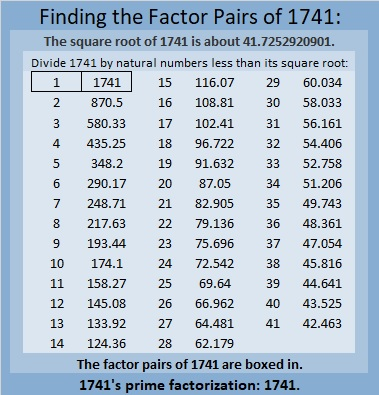How do we know that 1741 is a prime number? If 1741 were not a prime number, then it would be divisible by at least one prime number less than or equal to √1741. Since 1741 cannot be divided evenly by 2, 3, 5, 7, 11, 13, 17, 19, 23, 29, 31, 37, or 41, we know that 1741 is a prime number.

### More About the Number 1741:

1741 is the sum of two squares:
30² + 29² = 1741.

Here’s another way we know that 1741 is a prime number: Since its last two digits divided by 4 leave a remainder of 1, and 30² + 29² = 1741 with 30 and 29 having no common prime factors, 1741 will be prime unless it is divisible by a prime number Pythagorean triple hypotenuse less than or equal to √1741. Since 1741 is not divisible by 5, 13, 17, 29, 37, or 41, we know that 1741 is a prime number.

Since 29 and 30 are consecutive numbers, we have another fun fact: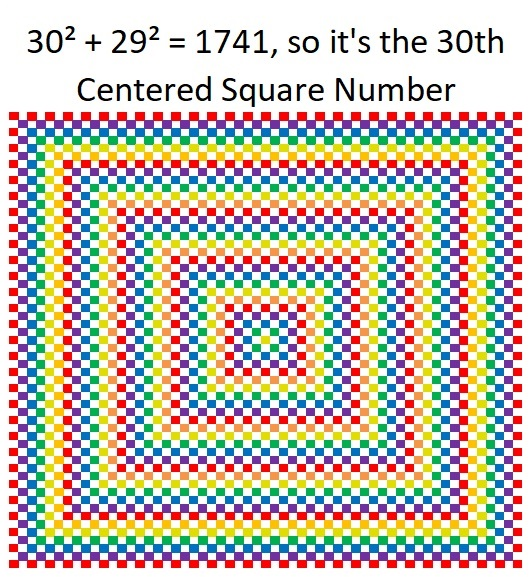And 1741 is the hypotenuse of a Pythagorean triple:
59-1740-1741, calculated from 30² – 29², 2(30)(29), 30² + 29².

1741 is also the difference of two consecutive squares:
871² – 870² = 1741.

# 1625 is a Centered Square Number

### Today’s Puzzle:

Because 1625 is the 29th centered square number, it is one more than four times the 28th triangular number. Can you draw lines on the graphic below separating out one tiny square and dividing the rest of the graphic into four equal triangles each with a base of 28 tiny squares?### Factors of 1625:

• 1625 is a composite number.
• Prime factorization: 1625 = 5 × 5 × 5 × 13, which can be written 1625 = 5³ × 13.
• 1625 has at least one exponent greater than 1 in its prime factorization so √1625 can be simplified. Taking the factor pair from the factor pair table below with the largest square number factor, we get √1625 = (√25)(√65) = 5√65.
• The exponents in the prime factorization are 3 and 1. Adding one to each exponent and multiplying we get (3 + 1)(1 + 1) = 4 × 2 = 8. Therefore 1625 has exactly 8 factors.
• The factors of 1625 are outlined with their factor pairs in the graphic below.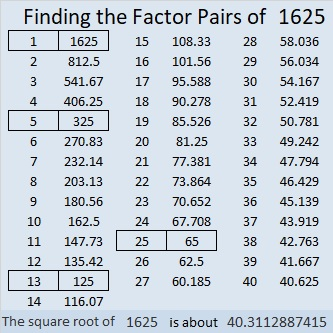### More about the Number 1625:

1625 is the sum of two squares FOUR different ways:
40² + 5² = 1625,
37² + 16² = 1625,
35² + 20² = 1625, and
29² + 28² = 1625.

1625 is the hypotenuse of TEN Pythagorean triples:
57-1624-1625, calculated from 29² – 28², 2(29)(28), 29² + 28²,
180-1615-1625, which is 5 times (36-323-325),
400-1575-1625, calculated from 2(40)(5), 40² – 5², 40² + 5²,
455-1560-1625, which is (7-24-25) times 65,
572-1521-1625, which is 13 times (44-117-125),
625-1500-1625, which is (5-12-13) times 125,
825-1400-1625, calculated from 35² – 20², 2(35)(20), 35² + 20²,
975-1300-1625, which is (3-4-5) times 325,
1020-1265-1625, which is 5 times (204-253-325), and
1113-1184-1625, calculated from 37² – 16², 2(37)(16), 37² + 16².

# 1405 is the Sum of Squares

I knew that 1405 was the sum of two consecutive squares, but OEIS.org let me know that it was the sum of even more consecutive squares, ELEVEN to be exact!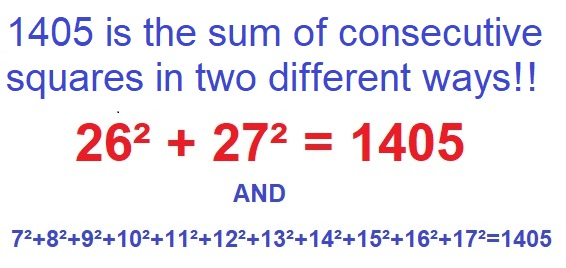Because it is the sum of the 26th and the 27th squares, 1405 is also the 27th centered square number. Here are 1405 tiny squares illustrating that fact:Here’s more about the number 1405:

• 1405 is a composite number.
• Prime factorization: 1405 = 5 × 281.
• 1405 has no exponents greater than 1 in its prime factorization, so √1405 cannot be simplified.
• The exponents in the prime factorization are 1, and 1. Adding one to each exponent and multiplying we get (1 + 1)(1 + 1) = 2 × 2 = 4. Therefore 1405 has exactly 4 factors.
• The factors of 1405 are outlined with their factor pair partners in the graphic below.I’ve mentioned one of these before, but 1405 is the sum of TWO squares in TWO ways:
27² + 26² = 1405
37² + 6² = 1405

1405 is also the hypotenuse of FOUR Pythagorean triples:
53-1404-1405 calculated from 27² – 26², 2(27)(26), 27² + 26²
444-1333-1405 calculated from 2(37)(6), 37² – 6², 37² + 6²
800-1155-1405 which is 5 times (160-231-281)
843-1124-1405 which is (3-4-5) times 281

# The factors of the hundred numbers just before 1201

I’ve made a simple chart of the numbers from 1101 to 1200, but it’s packed with great information. It gives the prime factorization of each of those numbers and how many factors each of those numbers have. The numbers written with a pinkish hue are the ones whose square roots can be simplified. Notice that each of those numbers has an exponent in its prime factorization.I didn’t make a horserace from the amounts of factors this time because it isn’t a very close race. Nevertheless, you can guess which number appears most often in the “Amount of Factors columns” and see if your number would have won the race.

Now I’ll share some information about the next number, 1201. Notice the last entry in the chart above. It had so many factors that there weren’t very many left for 1201 to have. . .

• 1201 is a prime number.
• Prime factorization: 1201 is prime.
• The exponent of prime number 1201 is 1. Adding 1 to that exponent we get (1 + 1) = 2. Therefore 1201 has exactly 2 factors.
• Factors of 1201: 1, 1201
• Factor pairs: 1201 = 1 × 1201
• 1201 has no square factors that allow its square root to be simplified. √1201 ≈ 34.65545

How do we know that 1201 is a prime number? If 1201 were not a prime number, then it would be divisible by at least one prime number less than or equal to √1201 ≈ 34.7. Since 1201 cannot be divided evenly by 2, 3, 5, 7, 11, 13, 17, 19, 23, 29 or 31, we know that 1201 is a prime number.Even though it doesn’t have many factors, 1201 is still a fabulous number:

25² + 24² = 1201

1201 is the 25th Centered Square Number because 25² + 24² = 1201, and 24 and 25 are consecutive numbers:1201 is the hypotenuse of a primitive Pythagorean triple:
49-1200-1201 calculated from 25² – 24², 2(25)(24), 25² + 24²

Here’s another way we know that 1201 is a prime number: Since its last two digits divided by 4 leave a remainder of 1, and 25² + 24² = 1201 with 25 and 24 having no common prime factors, 1201 will be prime unless it is divisible by a prime number Pythagorean triple hypotenuse less than or equal to √1201 ≈ 34.7. Since 1201 is not divisible by 5, 13, 17, or 29, we know that 1201 is a prime number.

# Mathemagical Properties of 1105

1105 is the magic sum of a 13 × 13 magic square. Why?
Because 13×13 = 169 and 169×170÷2÷13 = 13×85 = 1105.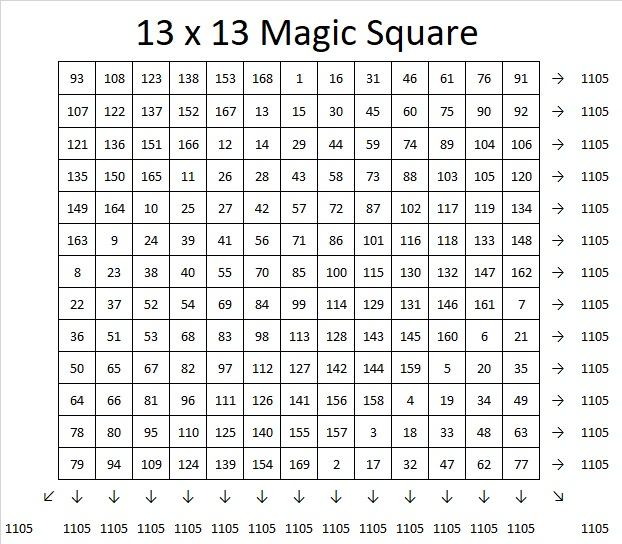If you follow the location of the numbers 1, 2, 3, 4, all the way to 169 in the magic square, you will see the pattern that I used to make that magic square. If you click on 10-factors-1102-1110  and go to the magic squares tab, you can use the same pattern or try another to create an 11 × 11, 13 × 13, or 15 × 15 magic square. The sums on the rows, columns, and diagonals will automatically populate as you write in the numbers so you can verify that you have indeed created a magic square.

1105 tiny squares can be made into a decagon so we say it is a decagonal number: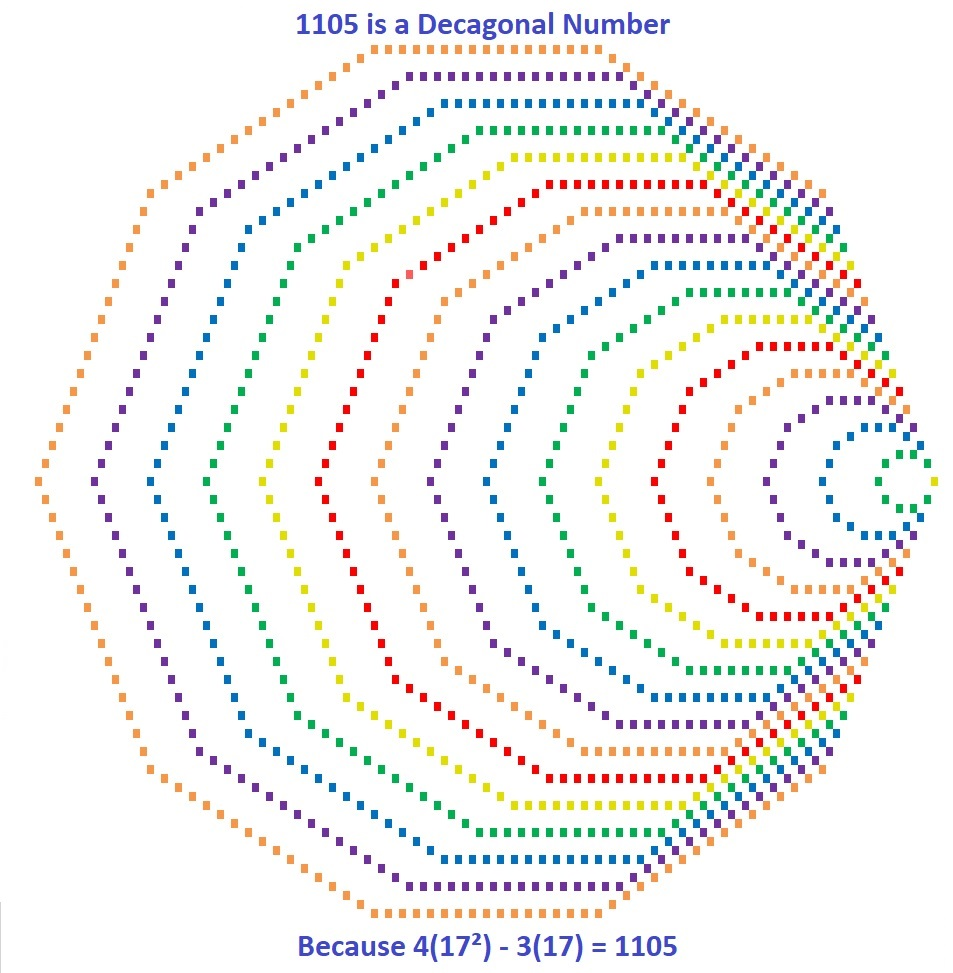Those 1105  tiny squares can also be arranged into a centered square: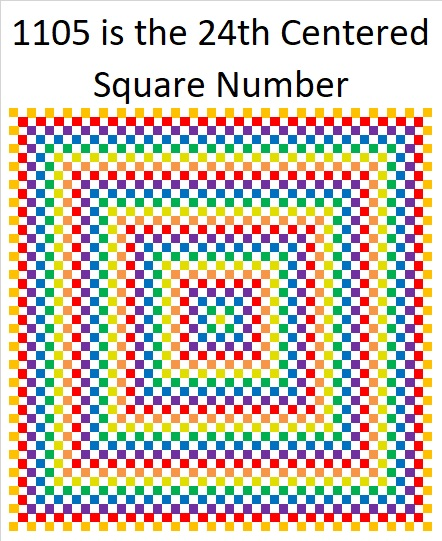Why is 1105 the 24th Centered Square Number? Because it is the sum of consecutive square numbers:
24² + 23² = 1105

But that’s not all! 1105 is the smallest number that is the sum of two squares FOUR different ways:

24² + 23² = 1105
31² + 12² = 1105
32² + 9² = 1105
33² + 4² = 1105

1105 is also the smallest number that is the hypotenuse of THIRTEEN different Pythagorean triples. Yes, THIRTEEN! (Seven was the most any previous number has had.) It is also the smallest number to have FOUR of its Pythagorean triplets be primitives (Those four are in blue type.):

47-1104-1105 calculated from 24² – 23², 2(24)(23), 24² + 23²
105-1100-1105 which is 5 times (21-220-221)
169-1092-1105 which is 13 times (13-84-85)
264-1073-1105 calculated from 2(33)(4), 33² – 4², 33² + 4²
272-1071-1105 which is 17 times (16-63-65)
425-1020-1105 which is (5-12-13) times 85
468-1001-1105 which is 13 times (36-77-85)
520-975-1105 which is (8-15-17) times 65
561-952-1105 which is 17 times (33-56-85)
576-943-1105 calculated from 2(32)(9), 32² – 9², 32² + 9²
663-884-1105 which is (3-4-5) times 221
700-855-1105 which is 5 times (140-171-221)
744-817-1105 calculated from 2(31)(12), 31² – 12², 31² + 12²

Why is it the hypotenuse more often than any previous number? Because of its factors! 1105 = 5 × 13 × 17, so it is the smallest number that is the product of THREE different Pythagorean hypotenuses.

It gets 1 triple for each of its three individual factors: 5, 13, 17, 2 triples for each of the three ways the factors can pair up with each other: 65, 85, 221, and four primitive triples for the one way they can all three be together: 1105. Thus it gets 2º×3 + 2¹×3 + 2²×1 = 3 + 6 + 4 = 13 triples.

Speaking of factors, let’s take a look at 1105’s factoring information:

• 1105 is a composite number.
• Prime factorization: 1105 = 5 × 13 × 17
• The exponents in the prime factorization are 1, 1, and 1. Adding one to each and multiplying we get (1 + 1)(1 + 1)(1 + 1) = 2 × 2 × 2 = 8. Therefore 1105 has exactly 8 factors.
• Factors of 1105: 1, 5, 13, 17, 65, 85, 221, 1105
• Factor pairs: 1105 = 1 × 1105, 5 × 221, 13 × 85, or 17 × 65
• 1105 has no square factors that allow its square root to be simplified. √1105 ≈ 33.24154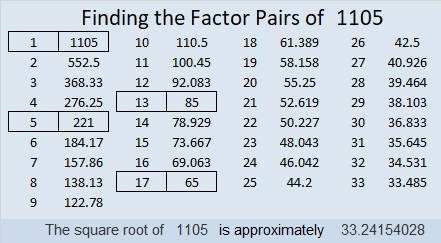1105 is also a palindrome in four different bases, and I also like the way it looks in base 8:
It’s 10001010001 in BASE 2 because 2¹º + 2⁶ + 2⁴ + 2º = 1105,
101101 in BASE 4 because 4⁵ + 4³ + 4² + 4º = 1105,
2121 in BASE 8 because 2(8³) + 1(8²) + 2(8) + 1(1) = 1105,
313 in BASE 19 because 3(19²) + 1(19) + 3(1) = 1105
1M1 in BASE 24 (M is 22 base 10) because 24² + 22(24) + 1 = 1105

Last, but certainly not least, you wouldn’t think 1105 is a prime number, but it is a pseudoprime: the second smallest Carmichael number. Only Carmichael number 561 is smaller than it is.

A Carmichael number is a composite number that behaves like a prime number by giving a false positive to all of certain quick prime number tests:
1105 passes the test p¹¹⁰⁵ Mod 1105 = p for all prime numbers p < 1105. Here is an image of my computer calculator showing 1105 passing the first five tests! Only a prime number should pass all these tests.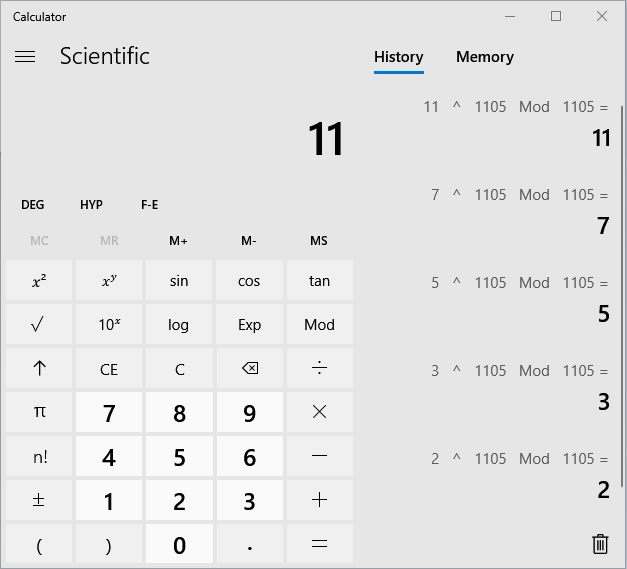1105 is indeed a number with amazing mathemagical properties!

# You’ll Be Impressed By 841’s Number Facts

841 is a very cool square number. 29 × 29 = 841. You can write it more compactly: 29² = 841. Here are some facts about this square number:

841 is the sum of the 29th and the 28th triangular numbers because 841 = 435 + 406.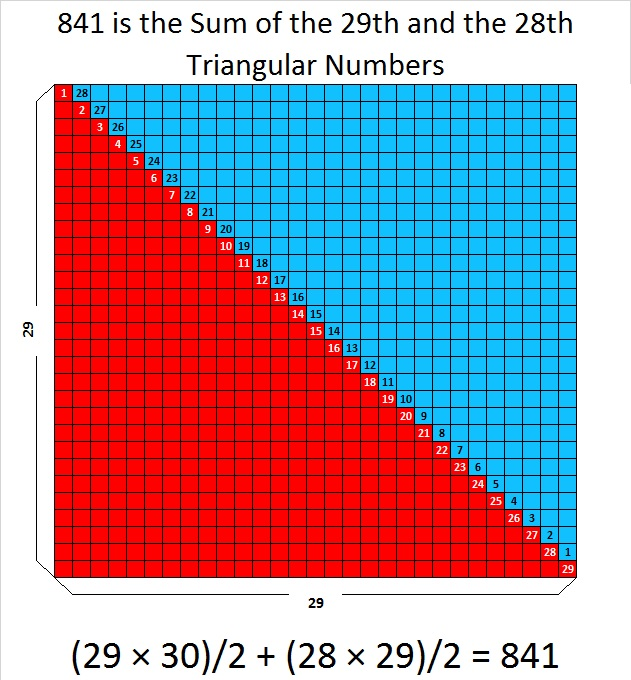What’s that you say? Every other square number can make a similar claim? Oh. … Wait a minute…Not EVERY square number can do that. One is a square number, and it’s NOT the sum of two triangular numbers. So there…

How about this… 841 is the sum of the first 29 odd numbers. That makes it the sum of all the odd numbers from 1 to 57. That’s impressive!What? All square numbers do that? Huh??? n² is always the sum of the first n odd numbers? Why’d you have to tell me that fact isn’t particularly unique either!

Okay…841 is an interesting number in a few other bases. Pay attention….  841 is

• 100 in BASE 29
• 121 in BASE 28
• 144 in BASE 27
• 169 in BASE 26
• 441 in BASE 14

Ha! You didn’t know that one! … Now don’t go spoiling my glee by telling me that those same square numbers show up for a few other square numbers, too. Don’t tell me that!

Give me one more chance to impress you….This square number, 841, is the sum of two consecutive square numbers, so 20² + 21² = 841 = 29². That hasn’t happened to a square number since 3² + 4² = 25 = 5². That makes 841 the 21st Centered Square Number but only the SECOND square number that is both kinds of squares! There may be an infinite number of squares that do the same thing, but it is still a fairly unique characteristic.

Here are a few other tidbits about 841:

841 is the sum of the nine prime numbers from 73 to 109. It is also the sum of three consecutive primes: 277 +  281 + 283 = 841

841 is the hypotenuse of TWO Pythagorean triples:

• 580-609-841 which is 29 times (20-21-29)
• 41-840-841 calculated from 21² – 20², 2(21)(20), 21² + 20²

841 Pythagorean triple Recursion: Hmm…How Quickly Can I Give You a Headache? Warning! Reading this set of bullet points might overload your brain:

• 5² + 2² = 29, so (2∙5∙2)² + (5² – 2²)² = (5² + 2²)² which means 20² + 21² = 29² = 841.
• 21² + 20² = 841, so (2∙21∙20)² + (21² – 20²)² = (21² + 20²)² which means 840² + 41² = 841² = (29²)² = 707281.
• 840² + 41² = 707281, so (2∙840∙41)² + (840² – 41²)² = (840² + 41²)² which means 68880² + 703919² = 707281² = ((29²)²)² = 500,246,412,961
• We could go on forever with even bigger powers of 29 …

We’ll finish with just some simple, easy-on-the-brain facts about 841:

29 is the tenth prime number. Its square, 841, is only the tenth number to have exactly three factors.

• 841 is a composite number.
• Prime factorization: 841 = 29²
• The exponent in the prime factorization is 2. Adding one we get (2 + 1) = 3. Therefore 841 has exactly 3 factors.
• Factors of 841: 1, 29, 841
• Factor pairs: 841 = 1 × 841 or 29 × 29
• 841 is a perfect square. √841 = 29I’m impressed by all this, even if you aren’t.

# 761 and Level 1

• 761 is a prime number.
• Prime factorization: 761 is prime.
• The exponent of prime number 761 is 1. Adding 1 to that exponent we get (1 + 1) = 2. Therefore 761 has exactly 2 factors.
• Factors of 761: 1, 761
• Factor pairs: 761 = 1 x 761
• 761 has no square factors that allow its square root to be simplified. √761 ≈ 27.586228.How do we know that 761 is a prime number? If 761 were not a prime number, then it would be divisible by at least one prime number less than or equal to √761 ≈ 27.6. Since 761 cannot be divided evenly by 2, 3, 5, 7, 11, 13, 17, 19, or 23, we know that 761 is a prime number.

There will be a lot about squares in today’s post, and all of the clues in today’s puzzle are perfect squares, too: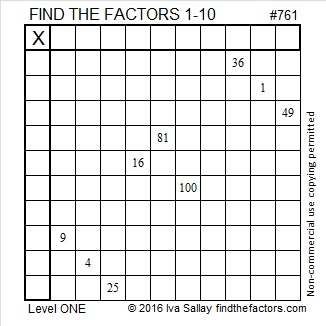Print the puzzles or type the solution on this excel file: 10 Factors 2016-02-04

—————————————

Here’s more about the number 761:

Because 761 is a prime number, there is only one way to write it as the sum of consecutive numbers: 380 + 381 = 761.

However, because it is a centered square number, 761 can be written as the sum of consecutive square numbers: 20² + 19² = 761.And because it is the sum of two consecutive square numbers, it is also the hypotenuse of a primitive Pythagorean triple: 39-760-761 which was calculated using 20² – 19², 2(20)(19), 20² + 19².

Notice also that 20 + 19 = 39, ⌊39²/2⌋ = 760, and ⌈39²/2⌉ = 761. (Those peculiar parenthesis mean⌊round down to the closest whole number⌋ and ⌈round up to the closest whole number⌉.)

In the 39 x 39 graphic shown above there are 760 white squares and 761 squares with some color. Thus, looking at that graphic is one way to look at the primitive Pythagorean triple 39-760-761.

All of that gives us another way to know that 761 is a prime number: Since its last two digits divided by 4 leave a remainder of 1, and 20² + 19² = 761 with 20 and 19 having no common prime factors, 761 will be prime unless it is divisible by a prime number Pythagorean hypotenuse less than or equal to √761 ≈ 27.6. Since 761 is not divisible by 5, 13, or 17, we know that 761 is a prime number.

761 can also be expressed as the sum of three squares TEN ways:

• 27² + 4² + 4² = 761
• 26² + 9² + 2² = 761
• 26² + 7² + 6² = 761
• 25² + 10² + 6² = 761
• 24² + 13² + 4² = 761
• 24² + 11² + 8² = 761
• 23² + 14² + 6² = 761
• 22² + 14² + 9² = 761
• 21² + 16² + 8² = 761
• 19² + 16² + 12² = 761

761 is a palindrome in two different bases:

• 535 BASE 12; note that 5(144) + 3(12) + 5(1) = 761.
• 1I1 BASE 20 (I = 18 base 10); note that 1(400) + 18(20) + 1(1) = 761.

—————————————# 685 Is the Sum of Two Squares Two Different Ways

Because both of its prime factors are hypotenuses of primitive Pythagorean triples, 685 is the hypotenuse of FOUR Pythagorean triples. Two are primitive; two are not:

• 37-684-685 which was calculated from 19² – 18², 2(19)(18), 19² + 18²
• 156-667-685 which was calculated from 2(26)(3), 26² – 3², 26² + 3²
• 411-548-685 (What factor of 685 is the greatest common factor of those 3 numbers?)
• 440-525-685 (and what is their greatest common factor?)

As you may have notice from those calculations, 685 is the sum of two squares two different ways:

• 19² + 18² = 685
• 26² + 3² = 685

685 is the 19th centered square number because 18 and 19 are consecutive numbers and 19² + 18² = 685. There are 685 small squares of various colors in this graphic.—————————————————————————————————

• 685 is a composite number.
• Prime factorization: 685 = 5 x 137
• The exponents in the prime factorization are 1 and 1. Adding one to each and multiplying we get (1 + 1)(1 + 1) = 2 x 2 = 4. Therefore 685 has exactly 4 factors.
• Factors of 685: 1, 5, 137, 685
• Factor pairs: 685 = 1 x 685 or 5 x 137
• 685 has no square factors that allow its square root to be simplified. √685 ≈ 26.172505.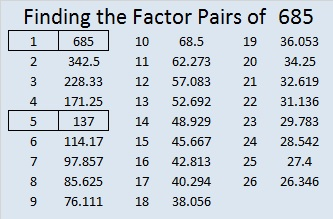—————————————————————————————————